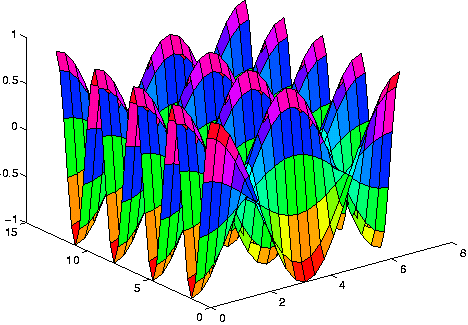# MATLAB

(redirected from .m)

## MATLAB

(mathematics, language, application)
A high-level language and interactive program from The MathWorks for numeric computation and visualisation. MATLAB supports numerical analysis, matrix computation, signal processing, linear algebra, statistics, Fourier analysis, filtering, optimisation and numerical integration. It can output two and three dimensional graphics and can be integrated with C, C++, Fortran, Java, COM and Microsoft Excel.

http://mathworks.com/products/matlab/.

## MATLAB

(MATrix LABoratory) A programming language for technical computing from The MathWorks, Natick, MA (www.mathworks.com). Used for a wide variety of scientific and engineering calculations, especially for automatic control and signal processing, MATLAB runs on Windows, Mac and a variety of Unix-based systems. Developed by Cleve Moler in the late 1970s and based on the original LINPACK and EISPACK FORTRAN libraries, it was initially used for factoring matrices and solving linear equations. Moler commercialized the product with two colleagues in 1984. MATLAB is also noted for its extensive graphics capabilities. The following MATLAB commands generate the 3D graph below:

```     x=(0:2*pi/20:2*pi)';
y=(0:4*pi/40:4*pi)';
[X,Y] = meshgrid(x,y);
z= cos(X).*cos(2*Y);
surf (x,y,z);
```

The Resulting GraphThis graph was computed from the lines of code above and is an example that might be used in multivariate calculus. (Image courtesy of Dr. Mark S. Gockenbach, Michigan Technological University, www.math.mtu.edu/~msgocken/intro/intro.html)
Copyright © 1981-2019 by The Computer Language Company Inc. All Rights reserved. THIS DEFINITION IS FOR PERSONAL USE ONLY. All other reproduction is strictly prohibited without permission from the publisher.
Site: Follow: Share:
Open / Close